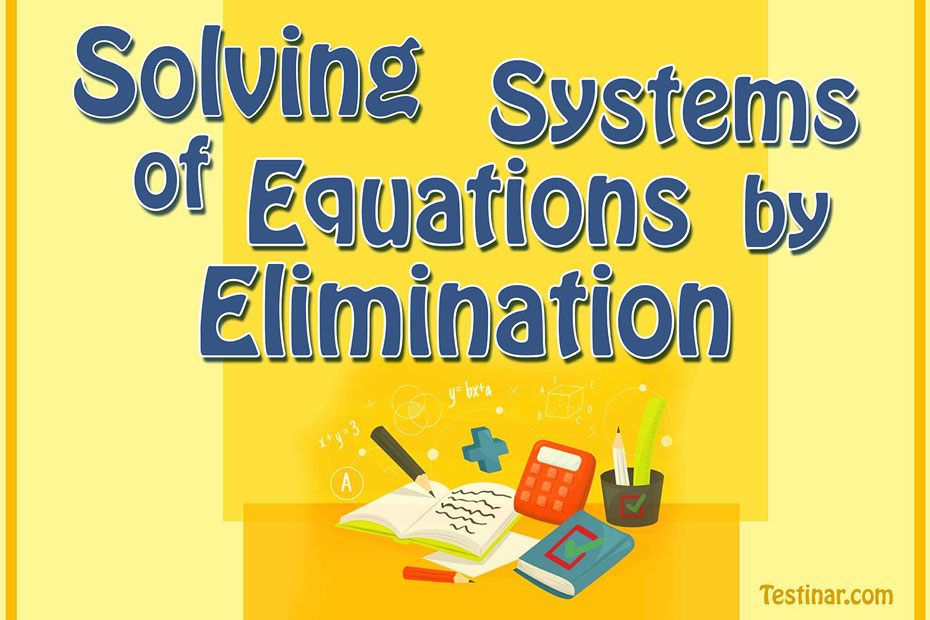## Solving Systems of Equations by Elimination

- The elimination method for solving systems of linear equations uses the addition property of equality. You can add the same value to each side of an equation.

Examole:

\cfrac{\begin{align}\ x\ +\ 2\ y\ =\ 6\\+ \ -\ x\ +\ y\ =\ 3\ \end{align}} {\begin{align}3\ y=9\\y=3\end{align}}\\ x\ +\ 6=6\\ x\ +\ 0

### New releases

$6.99$4.99

### Praxis Math Formulas

$6.99$5.99

### ISEE Middle Level Math Prep 2020

$20.99$15.99

### The Most Comprehensive CHSPE Math Preparation Bundle

$76.99$36.99